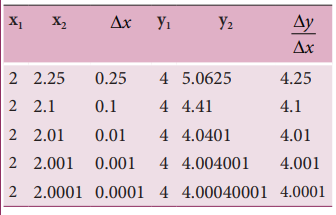Home | | Physics 11th std | Solved Example Problems for Differential Calculus

# Solved Example Problems for Differential Calculus

Physics : Kinematics : Differential Calculus

Solved Example Problems for Differential Calculus

### Example 2.18

Consider the function y = x 2 . Calculate the derivative dy/dx using the concept of limit.

### Solution

Let us take two points given byThese results are tabulated as shown below:From the above table, the following inferences can be made.### Example 2.19

Find the derivative with respect to t, of the function x= A0 + A1t + A2 t2 where A0, A1 and A2 are constants.

### Solution

Note that here the independent variable is ‘t’ and the dependent variable is ‘x’

The requived derivative is dx/dt = 0+ A1+2A2t

The second derivative is d2x/d2t = 2A2

Tags : Physics , 11th Physics : UNIT 2 : Kinematics
Study Material, Lecturing Notes, Assignment, Reference, Wiki description explanation, brief detail
11th Physics : UNIT 2 : Kinematics : Solved Example Problems for Differential Calculus | Physics